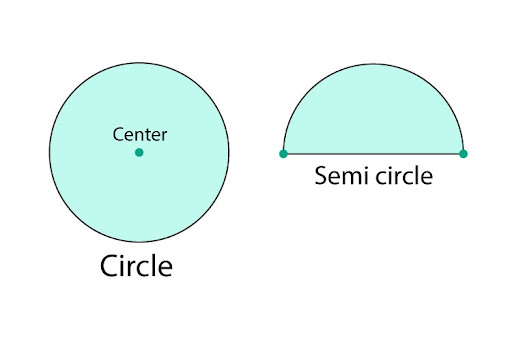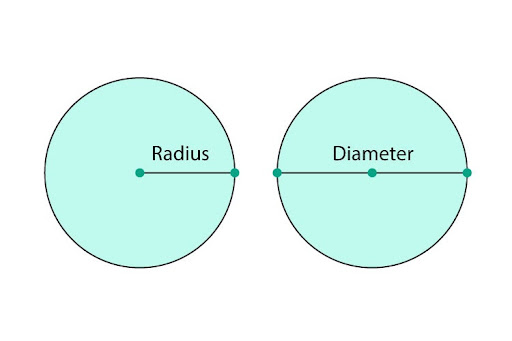# How can I work out the area of a semi-circle with a diameter of 12cm?

The question asks to calculate the area of a semicircle which is half a circle so the area of a semicircle is half the area of a circle.

Area of a semicircle = area of circle/2 or area of a circle = 2* area of semicircle.The formula for the area of a circle is pi times the radius squared:
Area of a circle = πr2   , where π = 22/7 or π = 3.142… and r = radius.
Thus, the area of a semicircle is half of pi times the radius squared.

Area of a semicircle = πr2 /2

The diameter is a straight line that runs through the center of the circle from one point on a circle to another. The radius is the line from the centre of the circle to any point on the circle.

Thus, the length of a radius is half the length of the diameter.